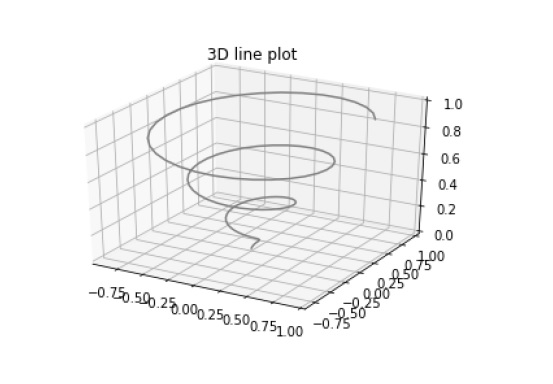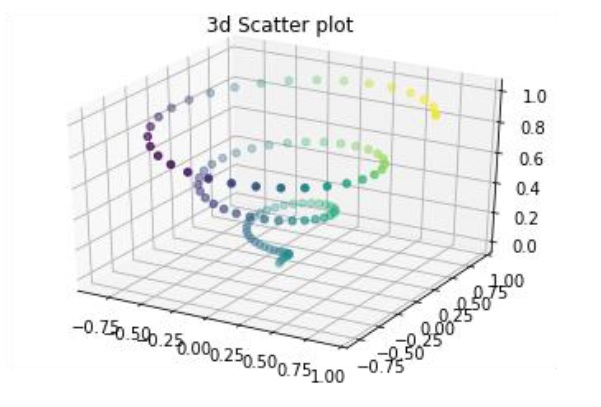# Matplotlib - Three-dimensional Plotting

Even though Matplotlib was initially designed with only two-dimensional plotting in mind, some three-dimensional plotting utilities were built on top of Matplotlib's two-dimensional display in later versions, to provide a set of tools for three-dimensional data visualization. Three-dimensional plots are enabled by importing the mplot3d toolkit, included with the Matplotlib package.

A three-dimensional axes can be created by passing the keyword projection='3d' to any of the normal axes creation routines.

```from mpl_toolkits import mplot3d
import numpy as np
import matplotlib.pyplot as plt
fig = plt.figure()
ax = plt.axes(projection='3d')
z = np.linspace(0, 1, 100)
x = z * np.sin(20 * z)
y = z * np.cos(20 * z)
ax.plot3D(x, y, z, 'gray')
ax.set_title('3D line plot')
plt.show()
```

We can now plot a variety of three-dimensional plot types. The most basic three-dimensional plot is a 3D line plot created from sets of (x, y, z) triples. This can be created using the ax.plot3D function.3D scatter plot is generated by using the ax.scatter3D function.

```from mpl_toolkits import mplot3d
import numpy as np
import matplotlib.pyplot as plt
fig = plt.figure()
ax = plt.axes(projection='3d')
z = np.linspace(0, 1, 100)
x = z * np.sin(20 * z)
y = z * np.cos(20 * z)
c = x + y
ax.scatter(x, y, z, c=c)
ax.set_title('3d Scatter plot')
plt.show()
```